# Basic Structural Model in SPSS AMOS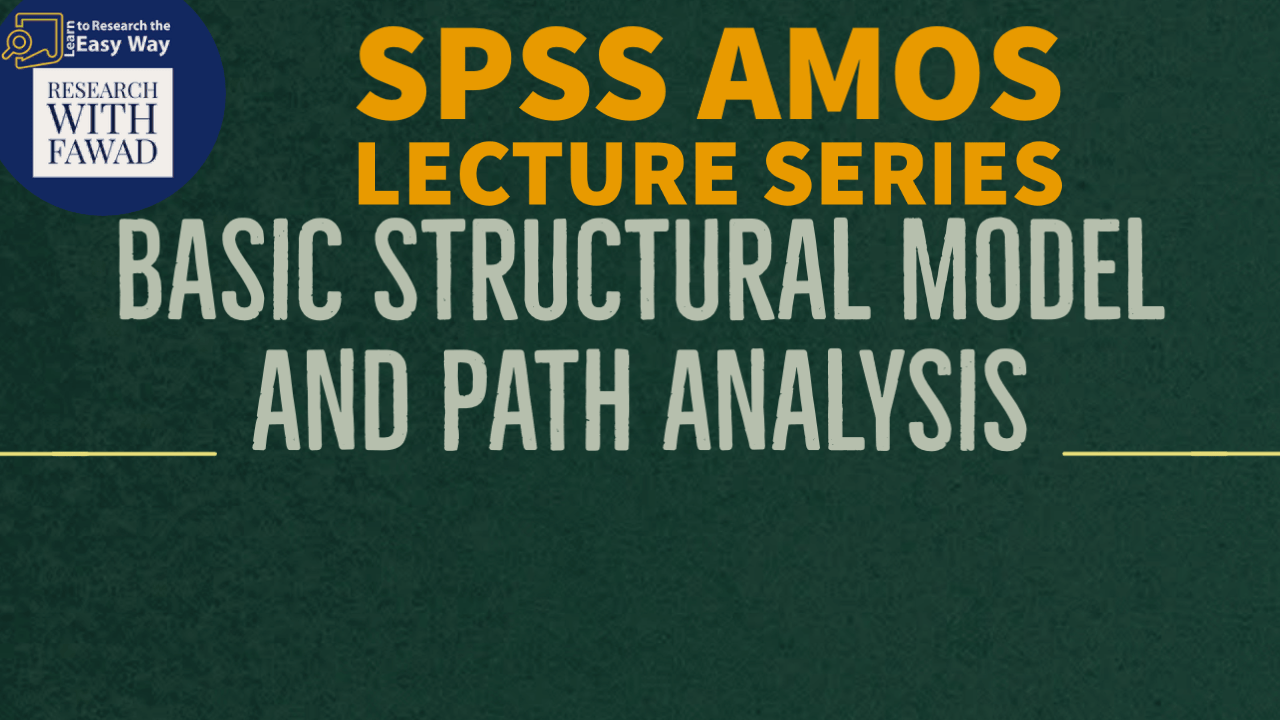### IBM SPSS AMOS Series

The Focus of the video tutorial is on Designing a very basic structural model in SPSS AMOS

## Path Analysis

• So far, we have talked only about the measurement model; now let’s talk about the structural model. The structural model’s focus is on examining the relationships between constructs. Hence, we will look at how independent constructs influence dependent constructs, or, in more complex models, how dependent variables influence other dependent variables.
• The first type of structural model we will examine is called a path analysis. In a path analysis, you are assessing only relationships between constructs (no measurement model items included). It is inappropriate to examine a path analysis until you have performed a measurement model analysis to determine if your measures are valid.
• Once the measurement model has been established, you can form composite variables for each construct. A path analysis examines the structural relationships between composite variables.
• How to form composite variables in SPSS. (Use Transform -> Computer Variable and take mean of the indicators for each construct).

## Proposed Path Model

The Following model is developed as part of understanding how to design, analyze, and interpret a path model in SPSS AMOS.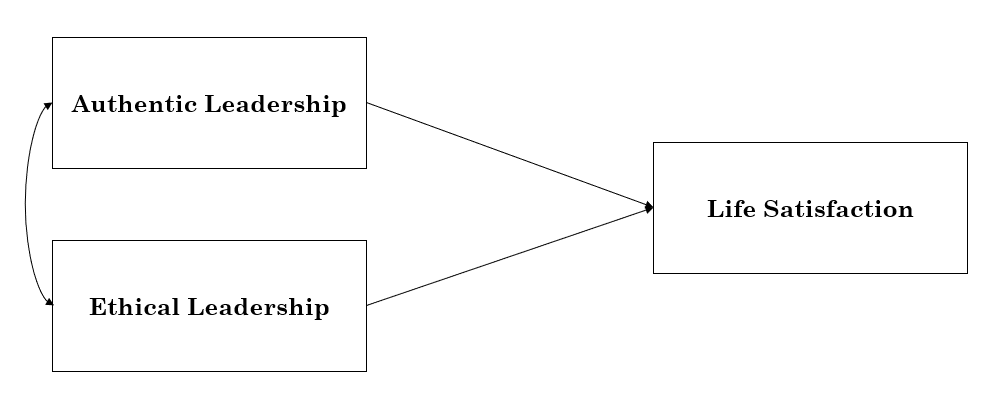## Building Path Model in SPSS AMOS

The tools used in the designing the model can Can be Selected from the Toolbar.

Step 1: Add the Observed Variable to the Canvas [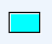]. In this case three Observed Variables (Al, BE, and LS) will be added to the path model.

Step 2: Draw Covariances [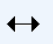] between the two predictors and Arrows [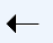] from the Two Predictors and the Outcome variable.

Step 3: Click the the Analysis Properties [  ], From the Output tab Select the following options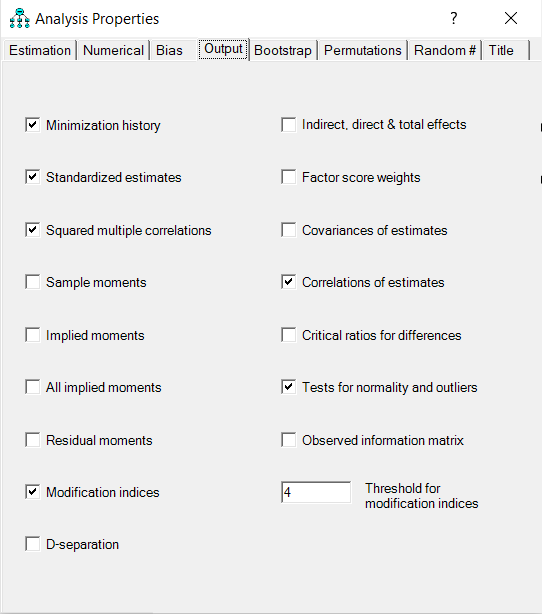Step 4: Now Run the Model by Clicking Calculate Estimates [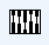]

## Analyzing the Output

Now that the model is estimated, now lets interpret the Model fit results and Estimates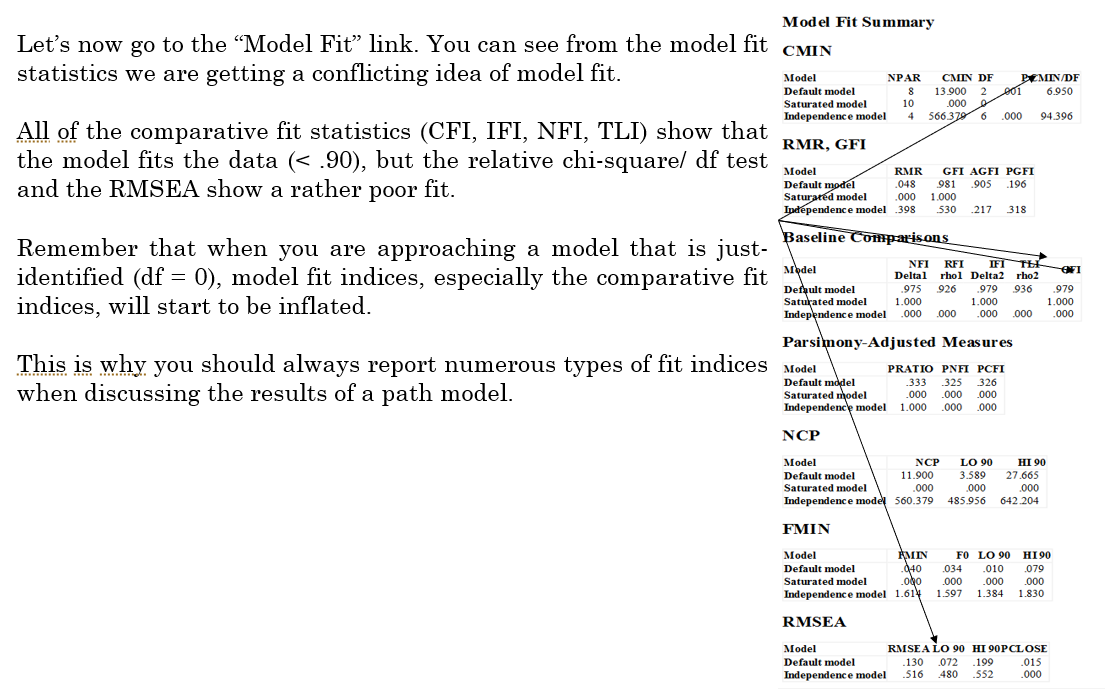Now that the model is estimated, now lets interpret the results.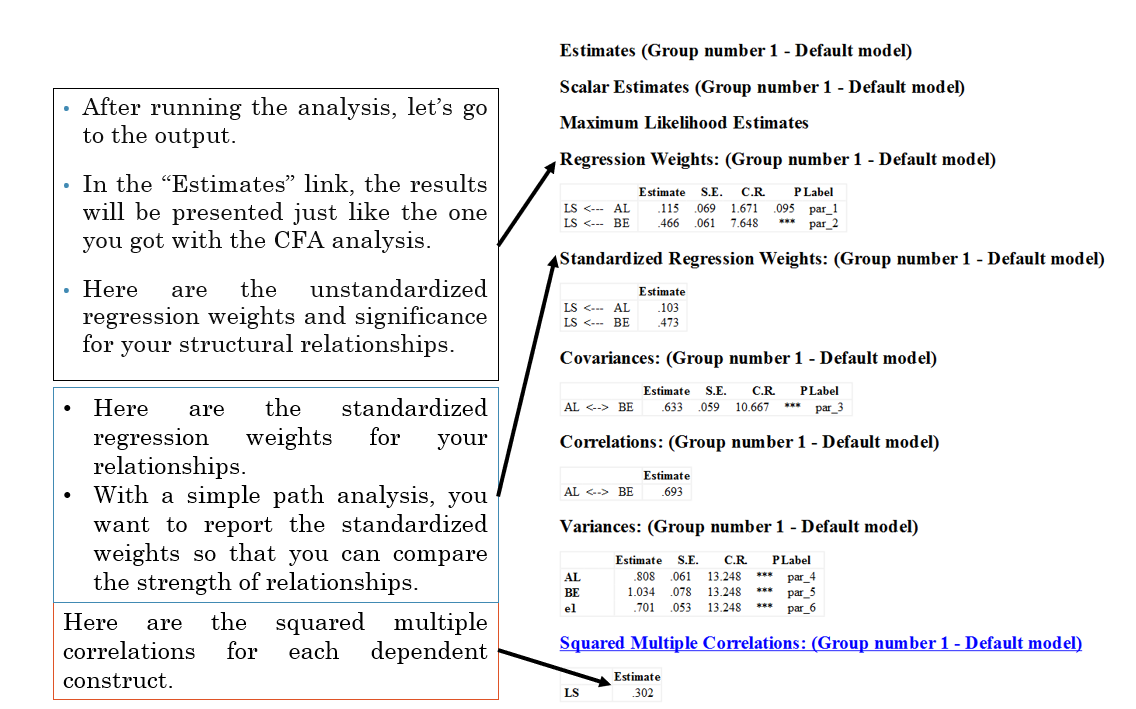## Reference

Collier, J. E. (2020). Applied structural equation modeling using AMOS: Basic to advanced techniques. Routledge.